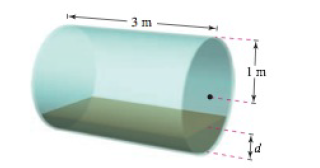Chapter 8.4, Problem 58E

Chapter
Section
Textbook Problem

# Volume The axis of a storage tank in the form of a right circular cylinder is horizontal (see figure). The radius and the length of the tank are 1 meter and 3 meters respectively.Determine the volume of fluid in the tank as a function of its depth d.Use a graphing utility to graph the function in part (a).Design a dip stick for the tank with markings of 1 4 , 1 2 , and 3 4 .Fluid is entering the tank at a rate of 1 4 cubic meter per minute. Determine the rate of change of the depth of the fluid as a function of its depth d.Use a graphing utility to graph the function in part (d). When will the rate of change of the depth be minimum? Does this agree with your intuition? Explain.

To determine

To calculate: The volume of the given storage tank.

Explanation

Given: The storage tank

Refer to the question for figure.

Calculation:

V=3.20d1(y1)2.dy=3[arcsin(d1)+(d1

### Still sussing out bartleby?

Check out a sample textbook solution.

See a sample solution

#### The Solution to Your Study Problems

Bartleby provides explanations to thousands of textbook problems written by our experts, many with advanced degrees!

Get Started

#### Find f. f(t) = 4/(1 + t2), f(1) = 0

Single Variable Calculus: Early Transcendentals, Volume I

#### In Exercises 21-24, find the distance between the given points. 24. (2, 1) and (10, 6)

Applied Calculus for the Managerial, Life, and Social Sciences: A Brief Approach

#### In problems 1-16, solve each equation. 4. Solve

Mathematical Applications for the Management, Life, and Social Sciences

#### Evaluate the integral. 11. 01/214x2dx

Single Variable Calculus: Early Transcendentals

#### Solve each equation. x4+81=0

Trigonometry (MindTap Course List)

#### In Exercises 116, determine whether the argument is valid. pq~pq

Finite Mathematics for the Managerial, Life, and Social Sciences

#### For f(x) = x3 + 7x, df = _____. a) (x3 + 7x) dx b) (3x2 + 7) dx c) 3x2 + 7 d) x3 + 7x + dx

Study Guide for Stewart's Single Variable Calculus: Early Transcendentals, 8th